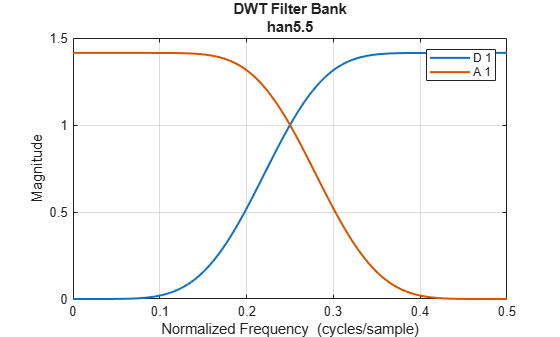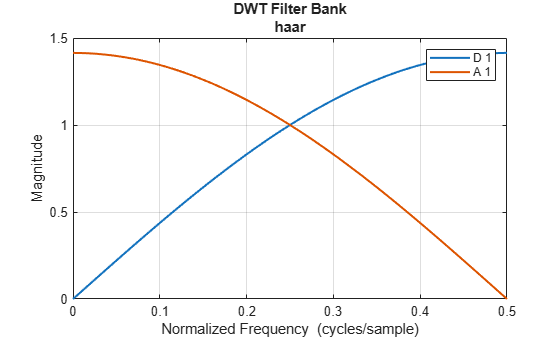# hanscalf

Han real orthogonal scaling filters with sum and linear-phase moments

Since R2022b

## Syntax

``scalf = hanscalf(wname)``

## Description

example

````scalf = hanscalf(wname)` returns the Han real-valued orthogonal scaling filter corresponding to `wname`.```

## Examples

collapse all

Obtain the scaling filter corresponding to the Han real orthogonal wavelet with five sum rules and five linear-phase moments.

`scalf = hanscalf("han5.5");`

Use `orthfilt` to obtain the scaling and wavelet filters corresponding to the wavelet.

`[LoD,HiD,LoR,HiR] = orthfilt(scalf);`

Confirm the filters form an orthonormal perfect reconstruction wavelet filter bank.

`[tf,checks] = isorthwfb(LoD)`
```tf = logical 1 ```
```checks=7×3 table Pass-Fail Maximum Error Test Tolerance _________ _____________ ______________ Equal-length filters pass 0 0 Even-length filters pass 0 0 Unit-norm filters pass 1.2168e-13 1.4901e-08 Filter sums pass 2.1645e-13 1.4901e-08 Even and odd downsampled sums pass 1.0836e-13 1.4901e-08 Zero autocorrelation at even lags pass 1.2484e-13 1.4901e-08 Zero crosscorrelation at even lags pass 2.1922e-17 1.4901e-08 ```

Create two discrete wavelet transform filter banks, one using the Han wavelet, and the other using the Haar wavelet. Specify a single level of decomposition for both filter banks. Plot the one-sided magnitude frequency responses of both filter banks. The Han wavelet has a larger frequency separation between the wavelet and scaling filters than the Haar wavelet.

```fbHan = dwtfilterbank(Wavelet="han5.5",Level=1); fbHaar = dwtfilterbank(Wavelet="haar",Level=1); freqz(fbHan)``````figure freqz(fbHaar)```## Input Arguments

collapse all

Han scaling filter, specified as `"hanSR.LP"`, where SR is the number of sum rules, and LP is the number of linear-phase moments. `wname` can be `"han2.3"`, `"han3.3"`, `"han4.5"`, or `"han5.5"`. For information on the filter properties, see Han Real Orthogonal Scaling Filters.

## Output Arguments

collapse all

Scaling filter corresponding to `wname`, returned as a vector. `scalf` should be used in conjunction with `orthfilt` to obtain scaling and wavelet filters with the proper normalization.

Data Types: `double`

collapse all

### Han Real Orthogonal Scaling Filters

Han filters are characterized by their order of sum rules, linear-phase moments, and phase. This table lists the filter specifications for the valid values of `wname`.

`wname`Order of Sum RulesNumber of Linear-Phase MomentsNormalized Variance of Filter Impulse ResponseFrequency Separation Between Scaling and Wavelet FilterLength
`"han2.3"` 230.4650.81566
`"han2.3"`230.4260.85408
`"han4.5"`450.4880.856310
`"han5.5"`550.5300.886714

Frequency separation is a number between 0 and 1, where 0 indicates the filters are perfectly matched and 1 indicates they are perfectly separated in frequency. As a point of reference, the Haar (`"db1"`) wavelet filter has the smallest normalized variance of all wavelet filters with 0.25 and poorest frequency separation with 0.666. An example of a scaling and wavelet filter pair with a relatively large frequency separation is the Fejér-Korovkin (`"fk22"`) 22-coefficient filter with a value of 0.9522.

 Han, Bin. “Wavelet Filter Banks.” In Framelets and Wavelets: Algorithms, Analysis, and Applications, 92–98. Applied and Numerical Harmonic Analysis. Cham, Switzerland: Birkhäuser, 2017. https://doi.org/10.1007/978-3-319-68530-4_2.